# R S Aggarwal Solutions for Class 11 Maths Chapter 30 Statistics Exercise 30C

Class 11 Chapter 30 – Statistics exercise 30C solutions are provided here. These solutions are outlined keeping in mind the current CBSE syllabus, hence possessing a great chance of scoring good marks in the exams. In this exercise, students will get a chance to solve miscellaneous word problems on the concept of statistics.

Class 11 Maths Chapter 30 Statistics Exercise 30C is based on the topic- Miscellaneous word problems. R S Aggarwal chapter 30 solutions for Class 11 are solved by the experts at BYJU’S and they have provided correct answers to all the questions. Students can download their pdf now and start practising.

## Download PDF of R S Aggarwal Solutions for Class 11 Maths Chapter 30 Statistics Exercise 30C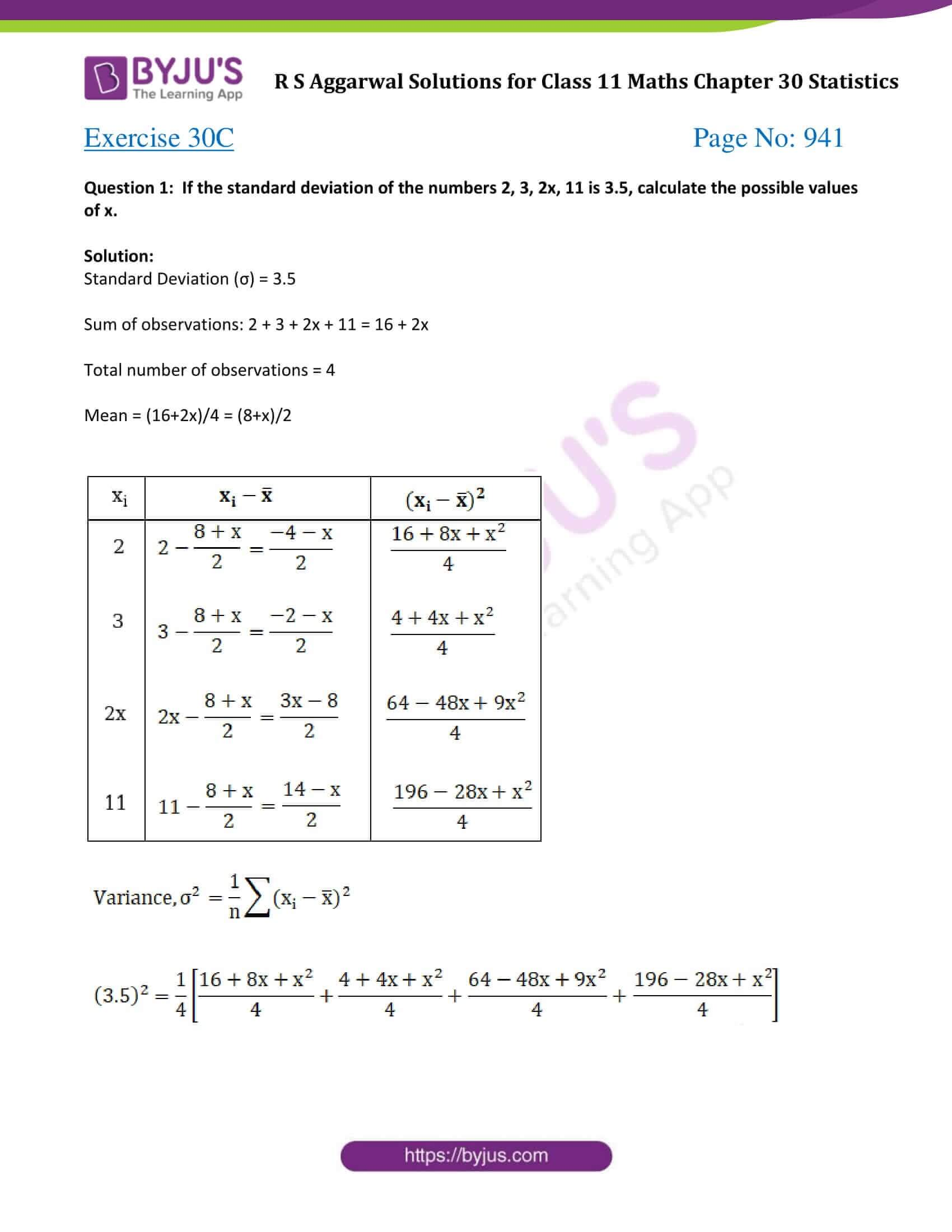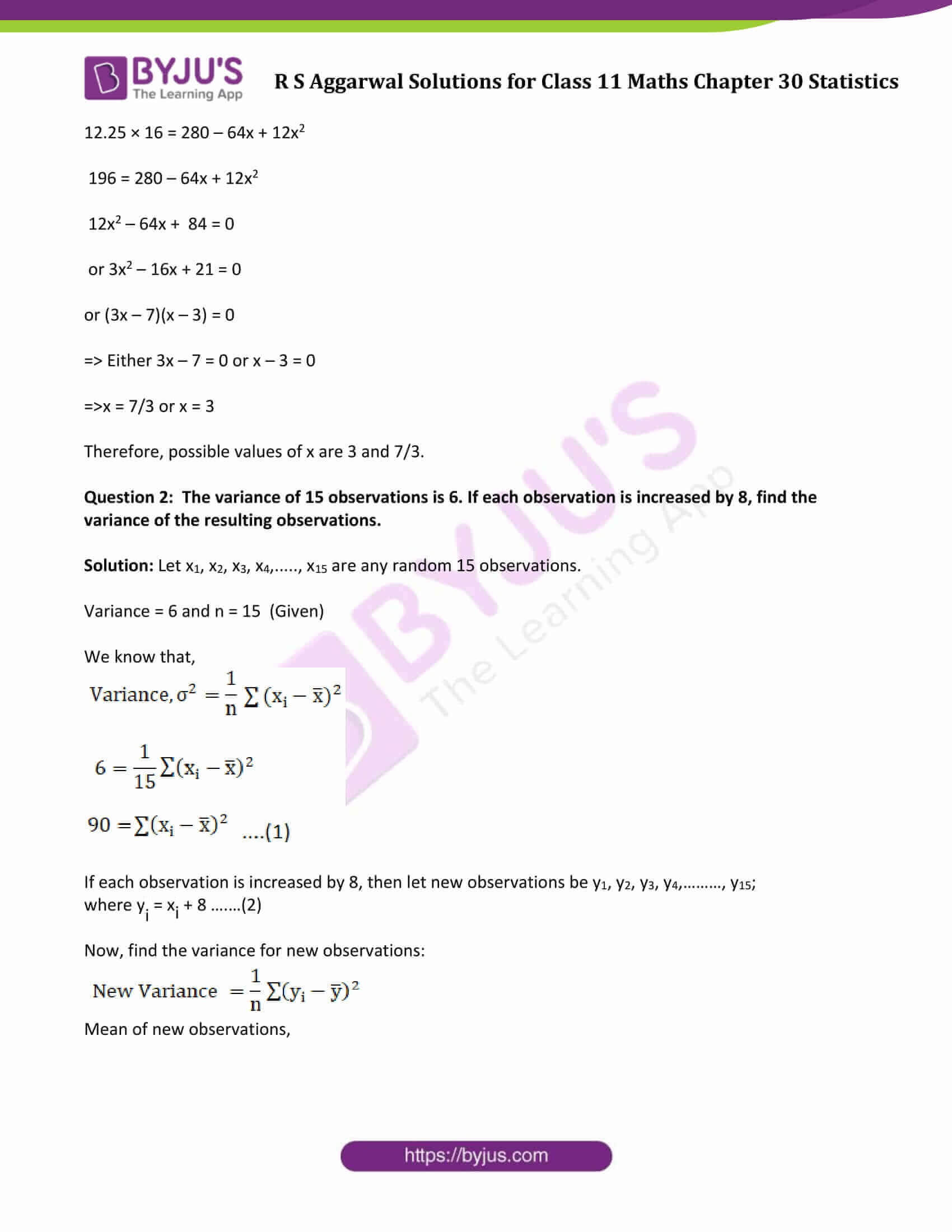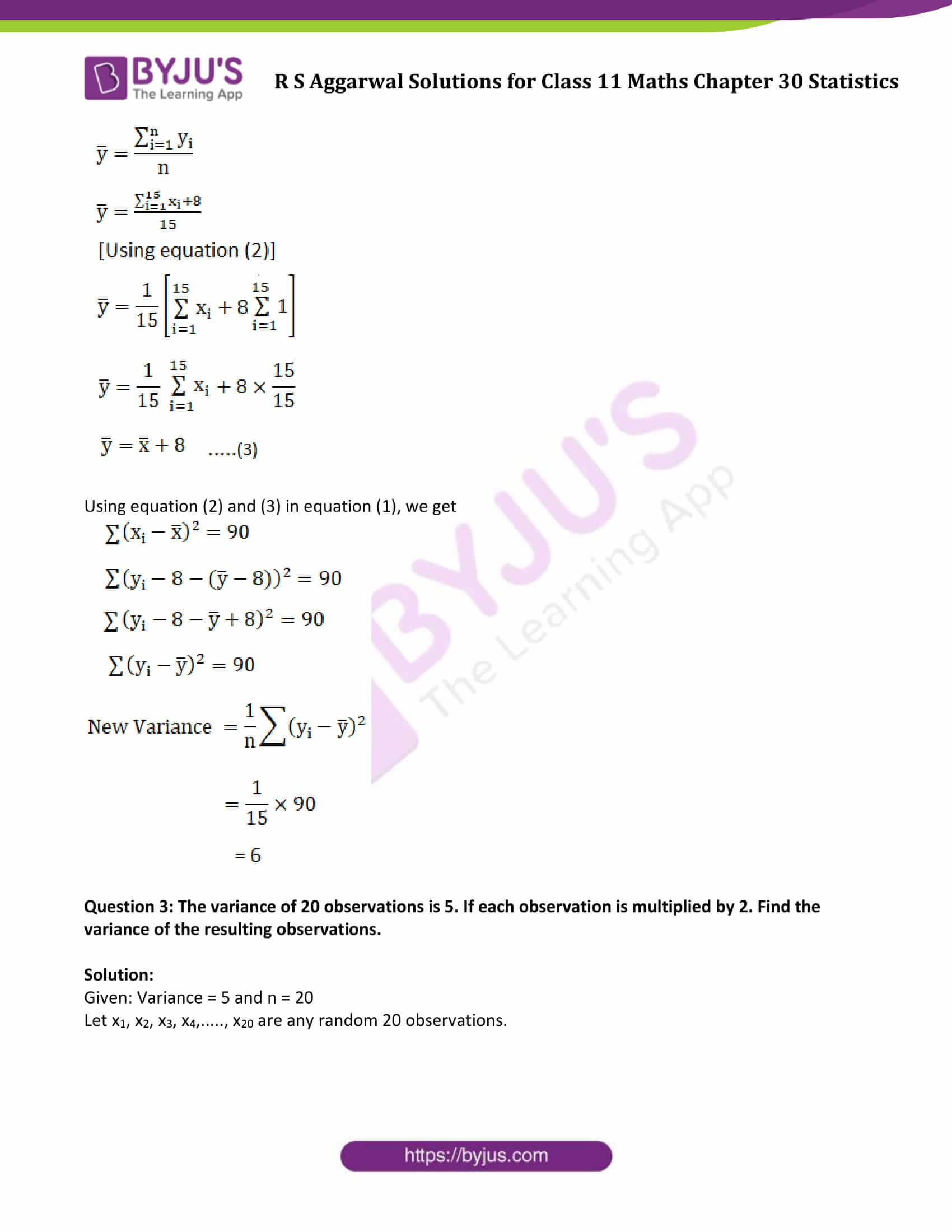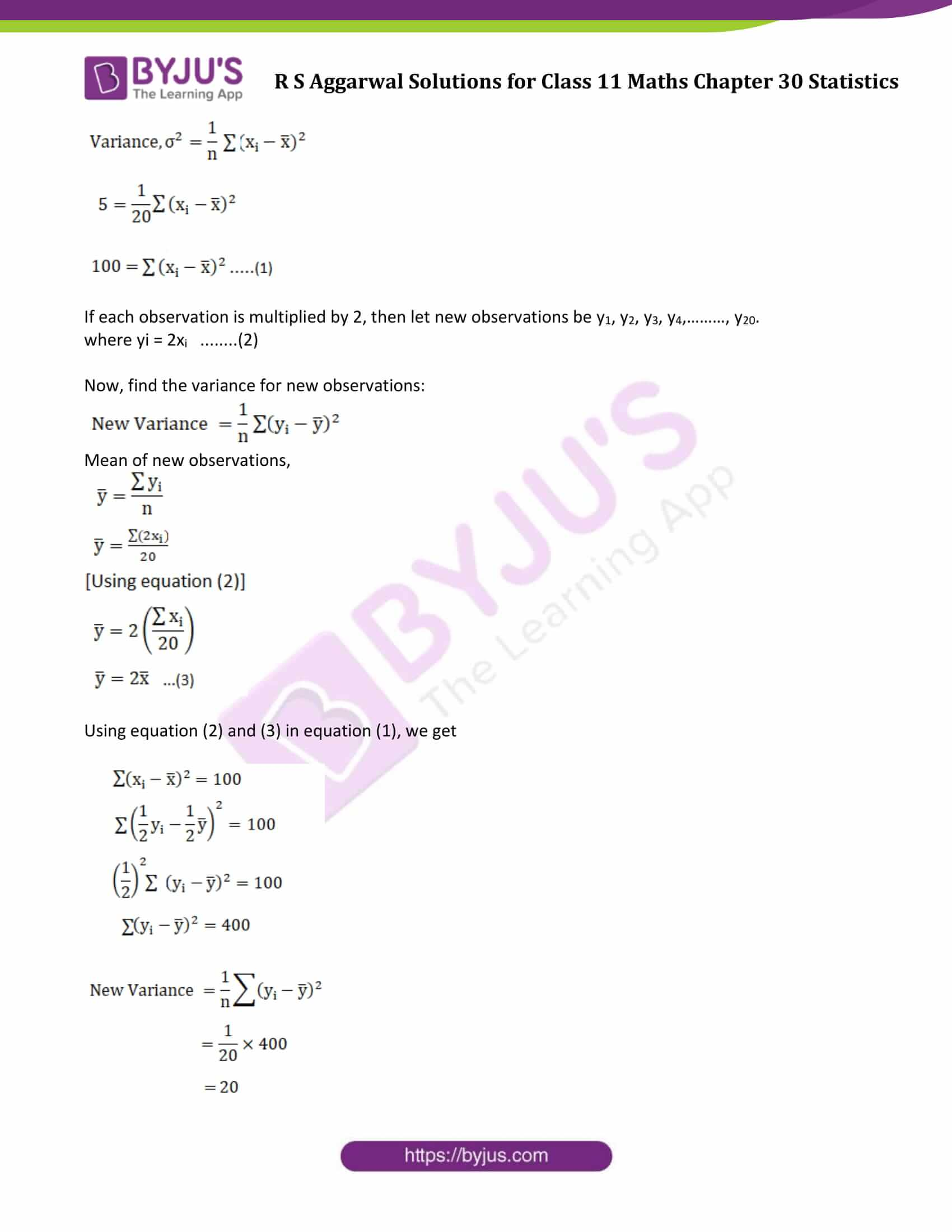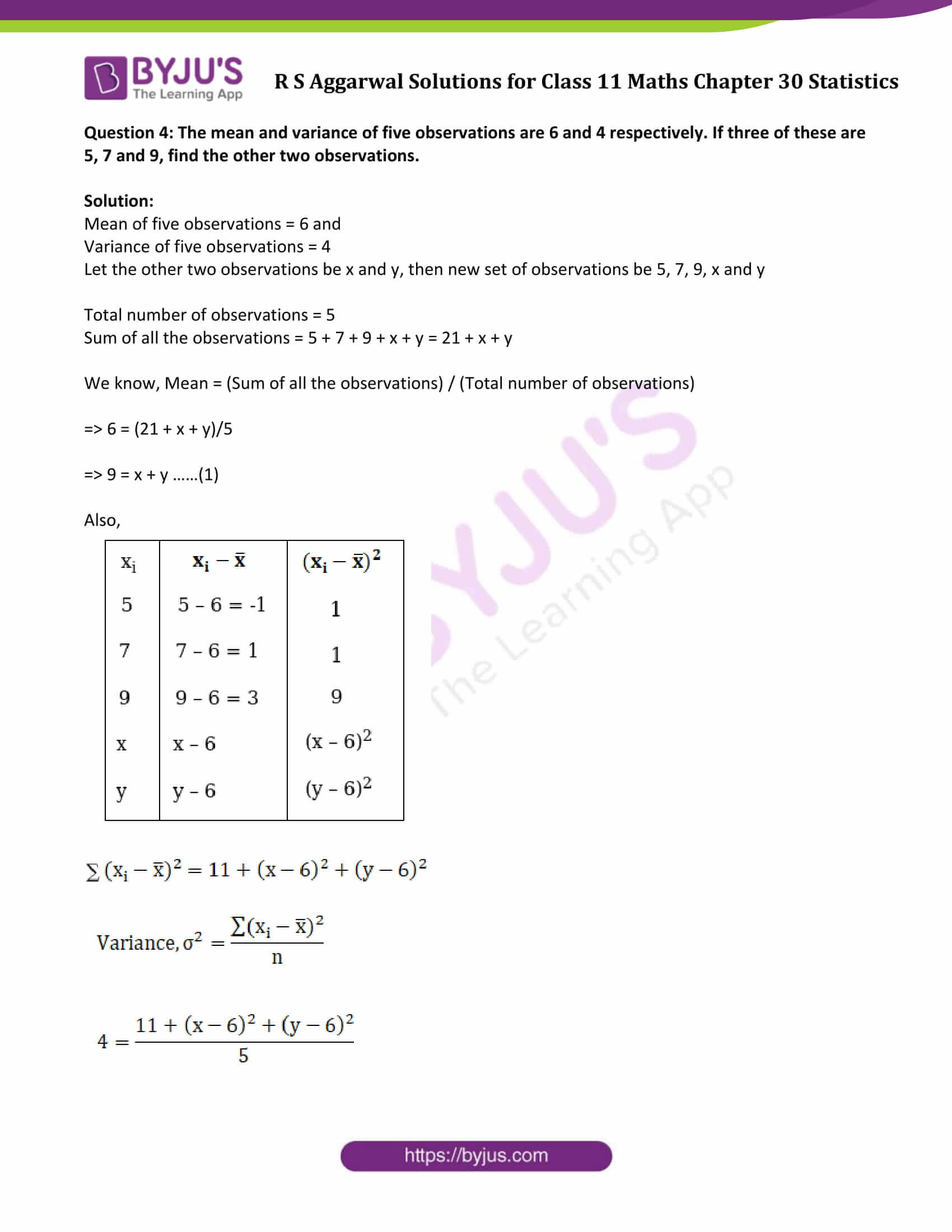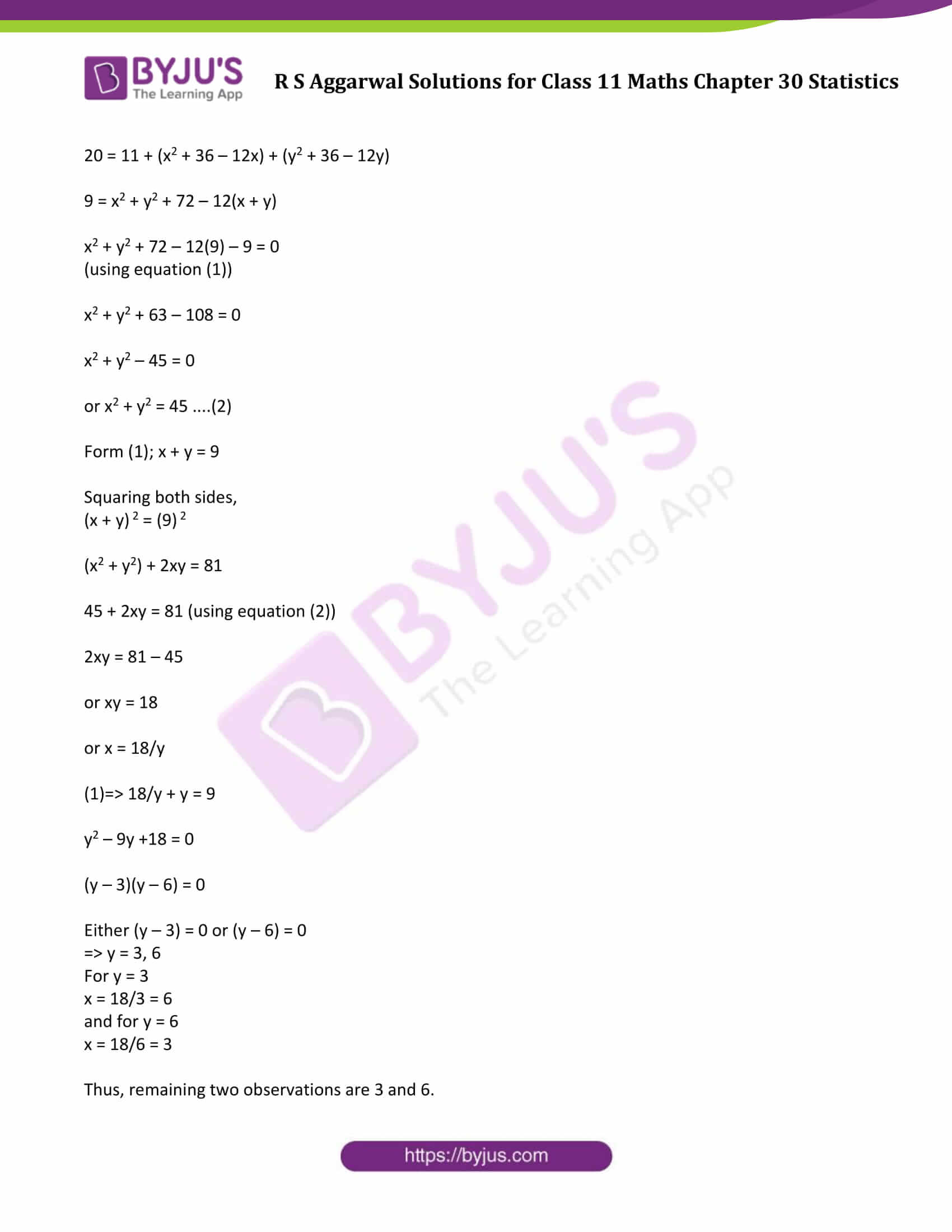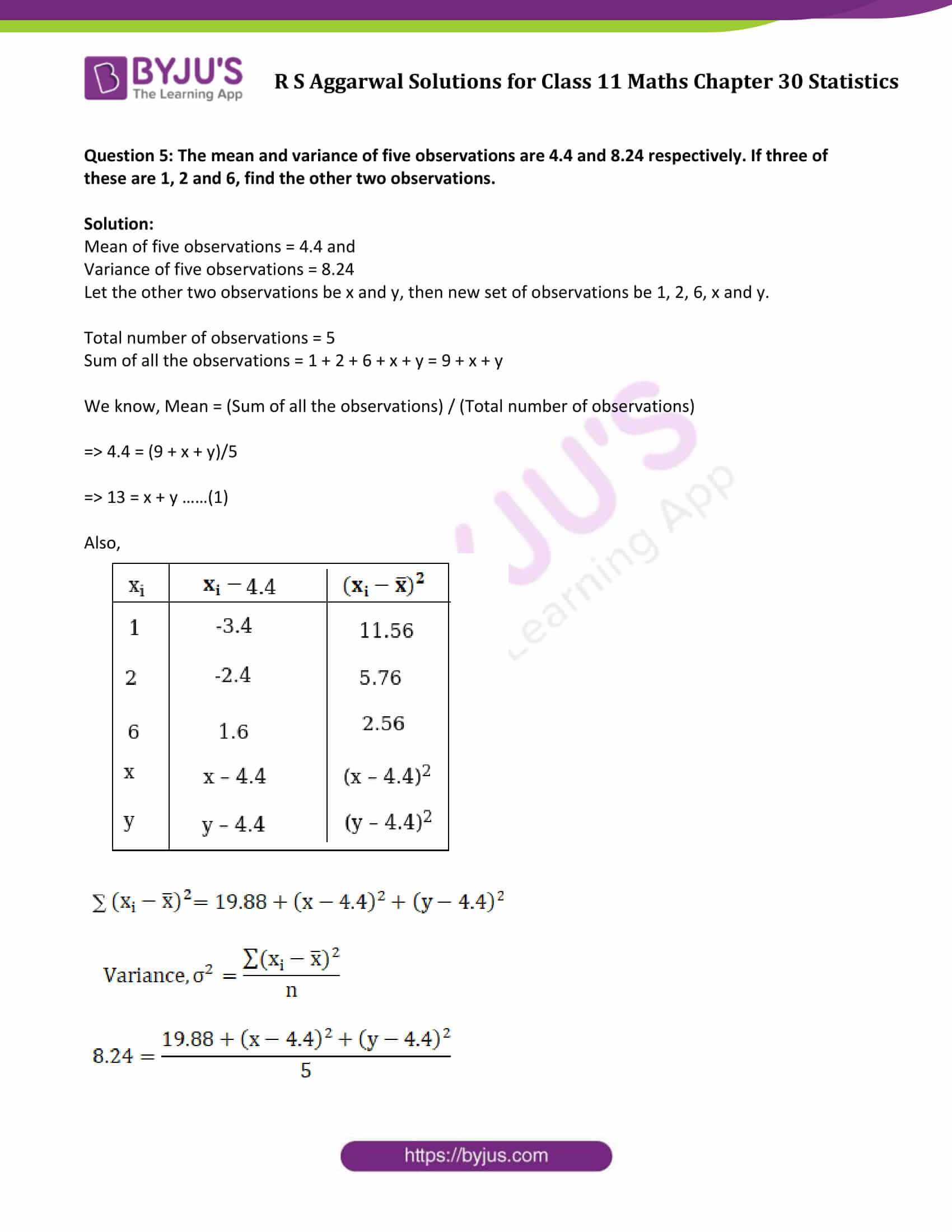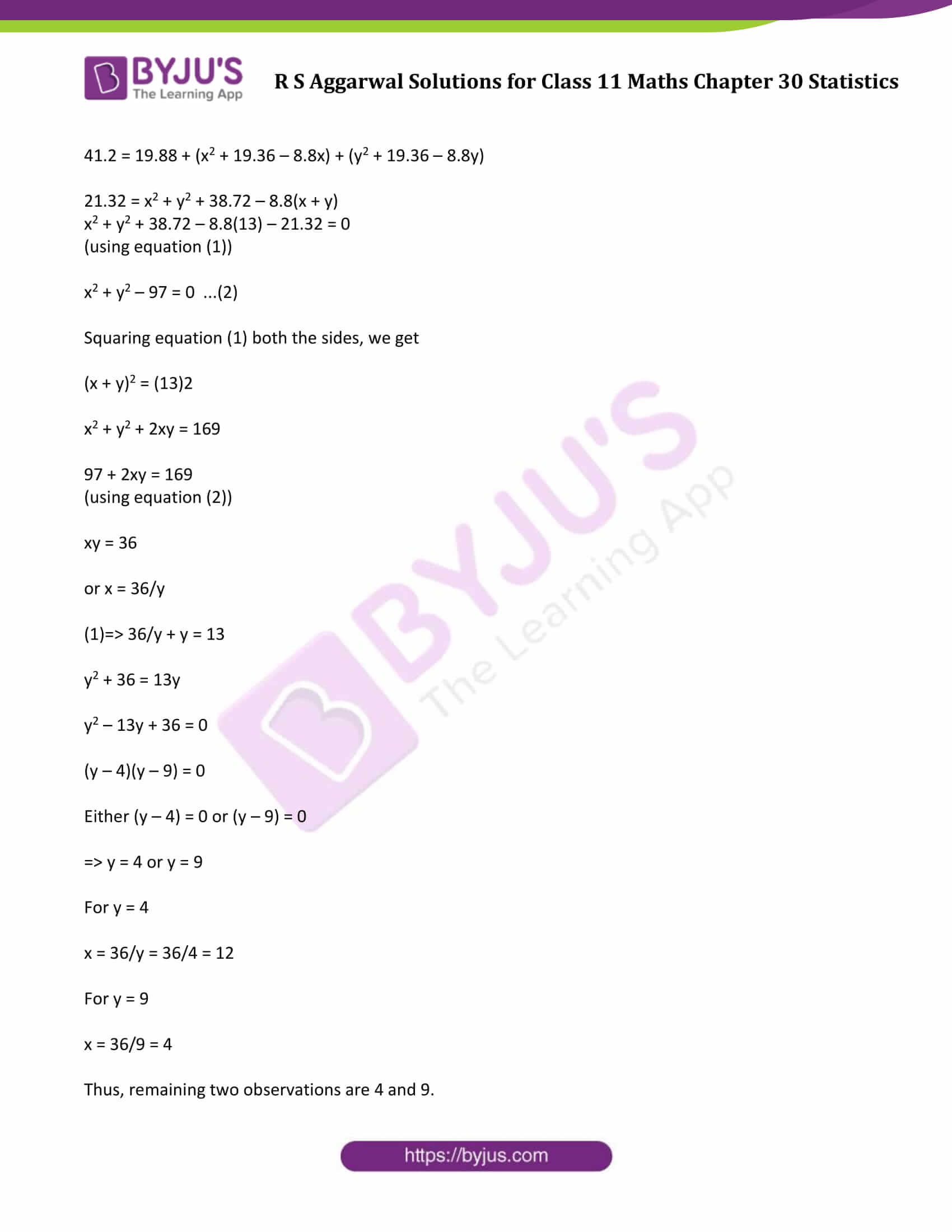### Access Answers to Maths R S Aggarwal Class 11 Chapter 30 Statistics Exercise 30C Page number 941

Question 1: If the standard deviation of the numbers 2, 3, 2x, 11 is 3.5, calculate the possible values of x.

Solution:

Standard Deviation (σ) = 3.5

Sum of observations: 2 + 3 + 2x + 11 = 16 + 2x

Total number of observations = 4

Mean = (16+2x)/4 = (8+x)/2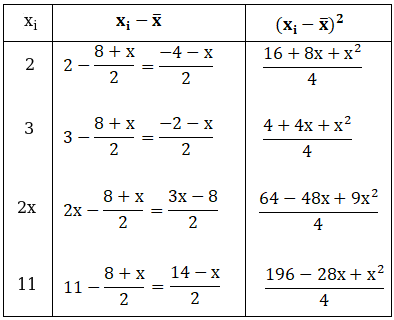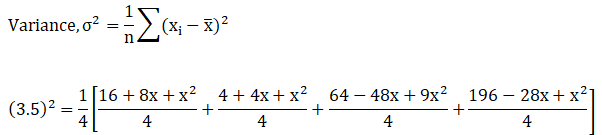12.25 × 16 = 280 – 64x + 12x2

196 = 280 – 64x + 12x2

12x2 – 64x + 84 = 0

or 3x2 – 16x + 21 = 0

or (3x – 7)(x – 3) = 0

=> Either 3x – 7 = 0 or x – 3 = 0

=>x = 7/3 or x = 3

Therefore, possible values of x are 3 and 7/3.

Question 2: The variance of 15 observations is 6. If each observation is increased by 8, find the variance of the resulting observations.

Solution: Let x1, x2, x3, x4,….., x15 are any random 15 observations.

Variance = 6 and n = 15 (Given)

We know that,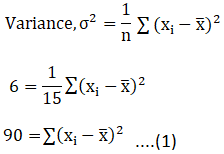If each observation is increased by 8, then let new observations be y1, y2, y3, y4,………, y15;

where yi = xi + 8 ….…(2)

Now, find the variance for new observations: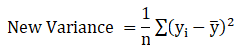Mean of new observations,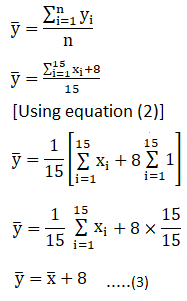Using equation (2) and (3) in equation (1), we get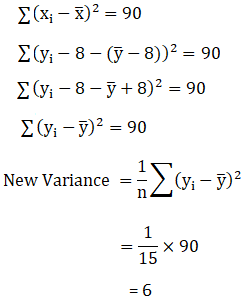Question 3: The variance of 20 observations is 5. If each observation is multiplied by 2. Find the variance of the resulting observations.

Solution:

Given: Variance = 5 and n = 20

Let x1, x2, x3, x4,….., x20 are any random 20 observations.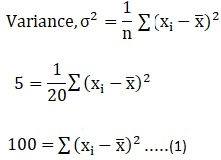If each observation is multiplied by 2, then let new observations be y1, y2, y3, y4,………, y20.

where yi = 2xi ……..(2)

Now, find the variance for new observations:Mean of new observations,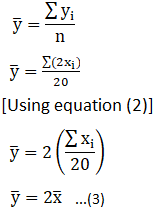Using equation (2) and (3) in equation (1), we get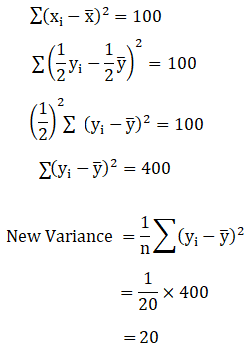Question 4: The mean and variance of five observations are 6 and 4 respectively. If three of these are 5, 7 and 9, find the other two observations.

Solution:

Mean of five observations = 6 and

Variance of five observations = 4

Let the other two observations be x and y, then new set of observations be 5, 7, 9, x and y

Total number of observations = 5

Sum of all the observations = 5 + 7 + 9 + x + y = 21 + x + y

We know, Mean = (Sum of all the observations) / (Total number of observations)

=> 6 = (21 + x + y)/5

=> 9 = x + y ……(1)

Also,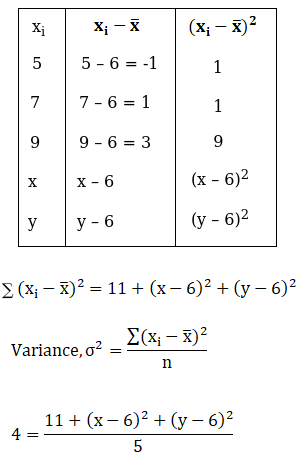20 = 11 + (x2 + 36 – 12x) + (y2 + 36 – 12y)

9 = x2 + y2 + 72 – 12(x + y)

x2 + y2 + 72 – 12(9) – 9 = 0

(using equation (1))

x2 + y2 + 63 – 108 = 0

x2 + y2 – 45 = 0

or x2 + y2 = 45 ….(2)

Form (1); x + y = 9

Squaring both sides,

(x + y) 2 = (9) 2

(x2 + y2) + 2xy = 81

45 + 2xy = 81 (using equation (2))

2xy = 81 – 45

or xy = 18

or x = 18/y

(1)=> 18/y + y = 9

y2 – 9y +18 = 0

(y – 3)(y – 6) = 0

Either (y – 3) = 0 or (y – 6) = 0

=> y = 3, 6

For y = 3

x = 18/3 = 6

and for y = 6

x = 18/6 = 3

Thus, remaining two observations are 3 and 6.

Question 5: The mean and variance of five observations are 4.4 and 8.24 respectively. If three of these are 1, 2 and 6, find the other two observations.

Solution:

Mean of five observations = 4.4 and

Variance of five observations = 8.24

Let the other two observations be x and y, then new set of observations be 1, 2, 6, x and y.

Total number of observations = 5

Sum of all the observations = 1 + 2 + 6 + x + y = 9 + x + y

We know, Mean = (Sum of all the observations) / (Total number of observations)

=> 4.4 = (9 + x + y)/5

=> 13 = x + y ……(1)

Also,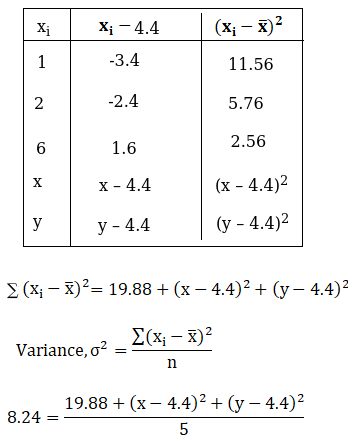41.2 = 19.88 + (x2 + 19.36 – 8.8x) + (y2 + 19.36 – 8.8y)

21.32 = x2 + y2 + 38.72 – 8.8(x + y)

x2 + y2 + 38.72 – 8.8(13) – 21.32 = 0

(using equation (1))

x2 + y2 – 97 = 0 …(2)

Squaring equation (1) both the sides, we get

(x + y)2 = (13)2

x2 + y2 + 2xy = 169

97 + 2xy = 169

(using equation (2))

xy = 36

or x = 36/y

(1)=> 36/y + y = 13

y2 + 36 = 13y

y2 – 13y + 36 = 0

(y – 4)(y – 9) = 0

Either (y – 4) = 0 or (y – 9) = 0

=> y = 4 or y = 9

For y = 4

x = 36/y = 36/4 = 12

For y = 9

x = 36/9 = 4

Thus, remaining two observations are 4 and 9.

## Access other exercise solutions of Class 11 Maths Chapter 30 Statistics

Exercise 30A Solutions

Exercise 30B Solutions

Exercise 30D Solutions